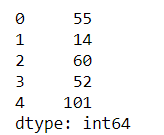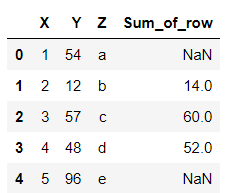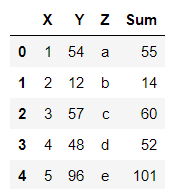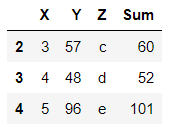# How to sum values of Pandas dataframe by rows?

• Last Updated : 26 Mar, 2021

While working on the python pandas module there may be a need, to sum up, the rows of a Dataframe. Below are the examples of summing the rows of a Dataframe. A Dataframe is a 2-dimensional data structure in form of a table with rows and columns. It can be created by loading the datasets from existing storage, storage can be SQL Database, CSV file, an Excel file, or from a python list or dictionary as well.

Pandas dataframe.sum() function returns the sum of the values for the requested axis.

Attention geek! Strengthen your foundations with the Python Programming Foundation Course and learn the basics.

To begin with, your interview preparations Enhance your Data Structures concepts with the Python DS Course. And to begin with your Machine Learning Journey, join the Machine Learning - Basic Level Course

Syntax: DataFrame.sum(axis)

Parameters:

• axis : {index (0), columns (1)}

Sum of each row:

`df.sum(axis=1)`

Example 1:

Summing all the rows of a Dataframe using the sum function and setting the axis value to 1 for summing up the row values and displaying the result as output.

## Python3

 `# importing pandas module as pd``import` `pandas as pd`` ` `# creating a dataframe using dictionary``df ``=` `pd.DataFrame({``'X'``:[``1``, ``2``, ``3``, ``4``, ``5``],``                   ``'Y'``:[``54``, ``12``, ``57``, ``48``, ``96``]})`` ` `# sum() method sums up the rows and columns of a dataframe``# axis = 1 sums up the rows``df ``=` `df.``sum``(axis ``=` `1``)``print``(df)`

Output :Sum of all the rows by index

Example 2:

Summing all the rows or some rows of the Dataframe as per requirement using loc function and the sum function and setting the axis to 1 for summing up rows. It sums up only the rows specified and puts NaN values in the remaining places.

## Python3

 `# importing pandas as pd``import` `pandas as pd`` ` `# creating the dataframe using pandas DataFrame``df ``=` `pd.DataFrame({``'X'``:[``1``, ``2``, ``3``, ``4``, ``5``],``                   ``'Y'``:[``54``, ``12``, ``57``, ``48``, ``96``],``                   ``'Z'``:[``'a'``, ``'b'``, ``'c'``, ``'d'``, ``'e'``]})`` ` `# df['column_name'] = df.loc[start_row_index:end_row_index,``# ['column1','column2']].sum(axis = 1)``# summing columns X and Y for row from 1 - 3``df[``'Sum_of_row'``] ``=` `df.loc[``1` `: ``3``,[``'X'` `, ``'Y'``]].``sum``(axis ``=` `1``)``print``(df)`

Output :Summing all rows from row 1 to 3

Example 3 :

Summing the rows using the eval function to evaluate the sum of the rows with the specified expression as a parameter.

## Python3

 `# importing pandas as pd``import` `pandas as pd`` ` `# creating the dataframe using pandas DataFrame``df ``=` `pd.DataFrame({``'X'``:[``1``, ``2``, ``3``, ``4``, ``5``],``                   ``'Y'``:[``54``, ``12``, ``57``, ``48``, ``96``],``                   ``'Z'``:[``'a'``, ``'b'``, ``'c'``, ``'d'``, ``'e'``]})`` ` `# eval('expression') calculates the sum of the specified columns of that row``df ``=` `df.``eval``(``'Sum = X + Y'``)``print``(df)`

Output :Sum of rows using the eval function

Example 4 :

Summing the rows using the eval function to evaluate the sum of the rows with specified rows using loc with the expression to calculate the sum as a parameter to eval function. It only returns the rows which are being specified in the loc and chops off the remaining.

## Python3

 `# importing pandas as pd``import` `pandas as pd`` ` `# creating the dataframe using pandas DataFrame``df ``=` `pd.DataFrame({``'X'``:[``1``, ``2``, ``3``, ``4``, ``5``],``                   ``'Y'``:[``54``, ``12``, ``57``, ``48``, ``96``],``                   ``'Z'``:[``'a'``, ``'b'``, ``'c'``, ``'d'``, ``'e'``]})`` ` `# eval('expression') calculates the sum``# of the specified columns of that row``# using loc for specified rows``df ``=` `df.loc[``2``:``4``].``eval``(``'Sum = X + Y'``)``display(df)`

Output :Summing specified rows only using eval

My Personal Notes arrow_drop_up